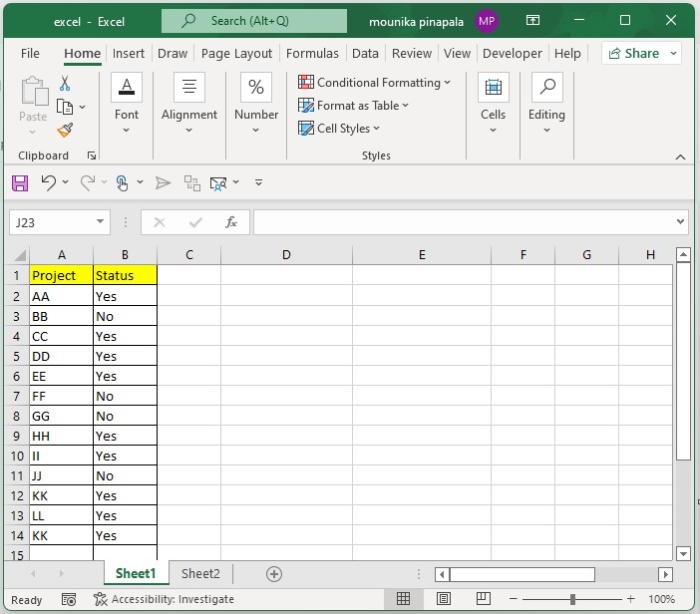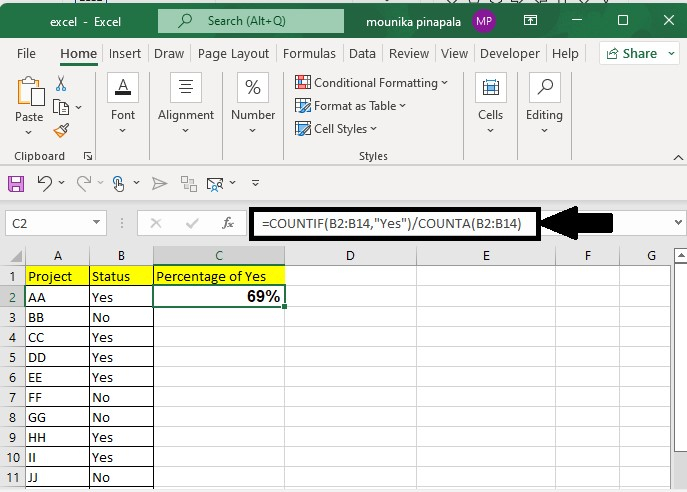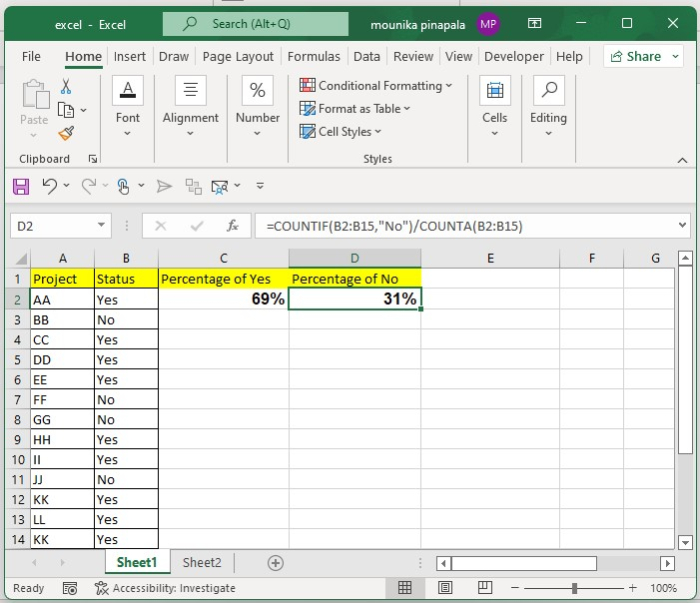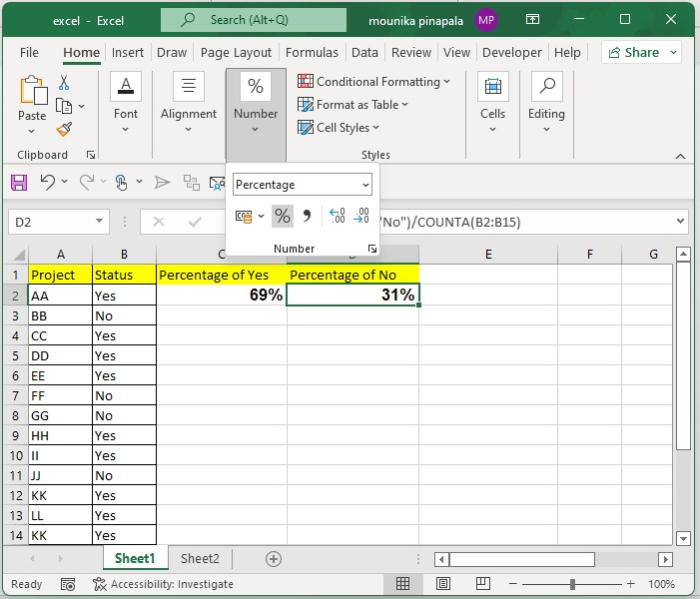# How to Calculate the Percentage of Yes and No from a List in Excel?

Suppose you have a list of "Yes" and "No" values in an Excel sheet and you need to calculate how many "Yes" and "No" are there in percentage terms. In this tutorial, we will show a simple method that you can use for this calculation.

## Step 1

Open a Microsoft Excel sheet, and enter the below status shown in the screenshot below for your reference. You can give status as per your wish.## Step 2

Now you need to enter the below given formula in the blank cell where you want the result of the yes percentage, and then press the Enter key, as shown in the following screenshot.

=COUNTIF(B2:B14,"Yes")/COUNTA(B2:B14)## Step 3

Now you need to enter the below given formula in the blank cell where you want the result of the no percentage, and then press enter key as shown in the below screenshot for your reference.

=COUNTIF(B2:B14,"No")/COUNTA(B2:B14)## Step 4

If you do not see the result in a percentage format. Then you need to go to Home and then under the number tab select the percentage symbol to get the result in the percentage format. Before that select the result and then click on the percentage symbol. Kindly find the below screenshot for your reference.In the above formula, B2:B14 is the list where you want to calculate the percentage of yes and no. Make sure you do not give any spaces in between the values, as the formula will give you the result as zero.

## Conclusion

In this tutorial, we used a simple example to show the easiest way to calculate the percentage of Yes and No from a list given in an Excel sheet.